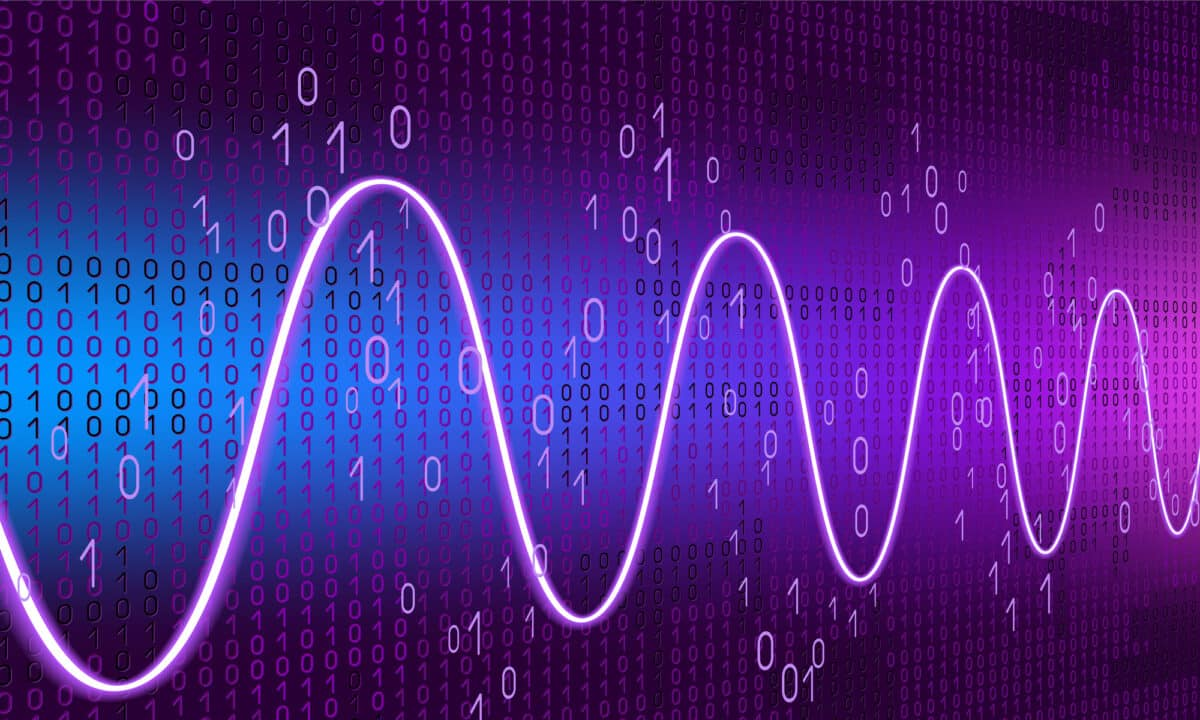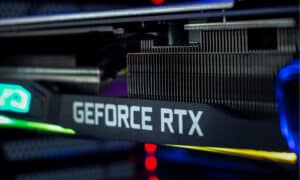Electrical engineering is all about signals. As such, the technology we rely on day in and day out is completely reliant on signals. Need proof? Try and change the channel on your TV when covering the transmitter on the remote. Need even more proof? Try and make a phone call when you’re out of range of a cell tower. An inconvenience that’s universally understood should be all the proof you need – signals help our technology work, and our technology helps make the world work.

However, it’s not always easy for analysts to visualize signal data in the easiest or most comprehensible way. From signals in continuous system theory to periodic and aperiodic signals to real and imaginary signals, sometimes it helps to visualize the data in a new or alternative way. This can make it easier to visualize and, as a result, easier to work with. The Hilbert transform is just one of many ways you can accomplish this.Though a tough concept to grasp, the Hilbert Transform is used in many applications, including signal processing.

## What is the Hilbert Transform?: Complete Explanation

In both math and signal processing, the Hilbert transform is a term that refers to the specific linear operator that takes a function of a real variable — or equation u(t) — and produces another function of a real variable. This is its Hilbert transform, or equation H(u)(t). For those who aren’t mathematicians (or signal processors), the concept can be a little difficult to grasp (after all, numbers and letters don’t mix very easily for many of us, and that’s the very foundation of a mathematical equation.) However, the Hilbert transform has immense importance because of its ability to make real functions applicable to analytic functions. This effectively gives the Hilbert transform a great number of different applications beyond simply being confined to a Hilbert transform table.

This is especially true of signal theory, where the Hilbert transform is also quite significant. Signal theory — also known as signal processing — is a component of electrical engineering. It involves the analysis, modification, and synthesis of audio or video signals and their respective scientific measurements. Techniques of signal theory help to strengthen signal transmissions, increase the efficiency and quality of stored signals, and highlight the key parts of a signal.

The Hilbert transform effectively shifts an equation’s negative frequency components by +90 degrees and an equation’s positive frequency components by –90 degrees. In other words, the Hilbert transform creates a 90-degree phase shift in data: sines become cosines, and cosines become sines. You can keep track of it all with a Hilbert transform table.

## The Hilbert Transform: An Exact Definition

Simply put, the Hilbert transform helps allow an analytic signal to form. This makes the Hilbert transform — and, by extension, the analytic signal — a vital part of signal theory. As such, the Hilbert transform is integral to the field of communications. Basically, the Hilbert transform is applied to real data that turns stationary data into specific complex data, effectively giving it a true, instantaneous amplitude in the form of waves. At the end of the day, it’s just another way to visualize the data you already have so you can see it in a new way (not unlike a Matlab or something similar). This is where a Hilbert transform table comes in handy.

## How Does the Hilbert Transform Work?

The concept works by representing pre-existing data in a different way for further analysis. It’s important to note that the Hilbert transform is not creating any new data or making any sort of prediction for what that data might look like in the future. It’s merely a way to add a new dimension to data you already have to examine it in a profound new way. Depending on the use, the Hilbert transform can potentially allow for new perspectives and new understandings of preexisting data. More than anything, the Hilbert transform works by organizing data more efficiently.

## How Do You Create the Hilbert Transform?

To create the Hilbert transform, you can look at its properties. These properties include:

• Boundedness
• Inverse transform
• Convolutions
• Complex structure
• Invariance
• Differentiation

If the data you’ve graphed abides by these properties, then you’re likely dealing with the Hilbert transform.

Alternatively, in the realm of signal processing, the Hilbert transform can be calculated in just a few simple steps:

• Use a calculator to determine the signal’s Fourier transform
• Reject any negative frequencies
• Determine the inverse Fourier transform

When done correctly, this creates a complex-valued signal that forms a Hilbert-transform pair with the data’s real and imaginary parts. (No Matlab needed!)

With that being said, many coding programs (such as Python or Matlab) also make it easy to create the Hilbert transform. Of course, Python (and other Python-like programs) are just further instances of the countless applications of the Hilbert transform.

## Where Did the Hilbert Transform Originate From?

The Hilbert transform originated in 1905 from mathematician David Hilbert’s work. This work came from a problem on analytic functions Bernhard Riemann brought to light. In the years since, this work became known as the Riemann–Hilbert problem. Hilbert’s work relied heavily on the work of another mathematician: George Boole.

In 1857, George Boole published a treatise titled “On the Comparison of Transcendent, with Certain Applications to the Theory of Definite Integrals.” This treatise, among other things, detailed how George Boole studied a rational function’s sum of residues. This is what’s referred to as Boole’s identity. Boole’s identity effectively helped facilitate the origination of the Hilbert transform.

## What Are the Applications of the Hilbert Transform

Because of its seemingly limitless applications, the Hilbert transform has often been called the most important operator in all of analytics. This is because it comes up in such a vast number of different contexts, with each one of these scenarios coalescing with each other in seemingly otherworldly ways. Some mathematicians and analysts have explained it like this: Dimension 1 has just one singular integral, and that’s the Hilbert transform. For this reason, the belief is that every significant analytic question can be reduced to this singular integral: the Hilbert transform. This means that, hypothetically, there are endless applications.

## Examples of the Hilbert Transform In the Real World

### Extracting the Envelope of a Signal

You can use the Hilbert transform to extract the envelope — also known as the magnitude — of an analytic signal. (The envelope of a signal also relates to its instantaneous phase and its frequency.)

### Creating an Analytic Signal

One of the many examples of the Hilbert transform in the real world is the creation of an analytic signal. When you’ve got a set of raw signal data and wish to analyze it in a new way, you can use the Hilbert transform to see those signals and that data differently.

### The Hilbert-Huang Transform

Another one of the many examples of the Hilbert transform in the real world is the similarly named Hilbert-Huang transform. This concept — coined by NASA — is a method used to break down a signal and its trend into its intrinsic mode functions (or IMF). The Hilbert-Huang transform works well with nonlinear data.

### Audio/Video Technology

In addition to these heady examples, there are also several much less complex uses for the Hilbert transform. These include:

• Use in high definition TV receivers
• Loudspeaker, surround sound acoustics, and mechanical vibration analysis for speakers
• Compression of audio and color images

## Theory of the Hilbert Transform FAQs (Frequently Asked Questions)

How do you find the Hilbert transform of a signal?

You don’t need to create a matlab to find the Hilbert transform of a signal. (You might not even need a calculator, either.) Basically, when all the positive frequency spectral components of a signal have shifted phase angles by -90 degrees and all the negative frequency spectral components shift phase angles by +90 degrees, you get the Hilbert transform of a signal.

What is the Hilbert transform used for?

Simply put, the Hilbert transform is a mathematical concept used to understand a spectral analysis’s minimum-phase response.

How do you calculate Hilbert transform?

The Hilbert transform isn’t necessarily something you can just key into a calculator. It really depends on how you want to use the Hilbert transform. For instance, in continuous system theory, H[g(t) + c] = gˆ(t) + cˆ = gˆ(t). (Continuous system theory involves frequencies where the input and the output signals are identical.)

What is Hilbert Transform of cos wt?

The Hilbert transform of cos wt is sinwt.

What is Hilbert transform DSP?

A Hilbert transform DSP is a digital signal processor that comprehends frequency signals and uses them to convey audio or visual information.

What is the difference between the Hilbert transform and the Fourier transform?

While they sound similar in name, the Hilbert transform and the Fourier transform serve different purposes altogether. Whereas the Hilbert transform is used to determine what a signal does in its frequency space, the Fourier transform is used to determine the frequency of a signal itself.

## More from History-Computer##### Nvidia RTX 3080 vs 3080-Ti: Full Comparison With Specs, Price, and More##### The 10 Largest Chip Manufacturers in the World and What They Do##### The 10 Largest and Most Important Battery Companies in the World
• comm.utoronot.ca Available here: https://www.comm.utoronto.ca/frank/notes/hilbert.pdf
• fuchs-braun Available here: http://www.fuchs-braun.com/media/d9140c7b3d5004fbffff8007fffffff0.pdf
• diva-portal Available here: https://www.diva-portal.org/smash/get/diva2:872439/FULLTEXT02.pdf
• tutorials point Available here: https://www.tutorialspoint.com/signals-and-systems-what-is-hilbert-transform
• math works Available here: https://www.mathworks.com/help/signal/ug/hilbert-transform.html
• dsp related Available here: https://www.dsprelated.com/freebooks/sasp/Hilbert_Transform_Design_Example.html
• fly lib Available here: https://flylib.com/books/en/2.729.1/why_care_about_the_hilbert_transform_.html
• comm.utoronot.ca Available here: https://www.comm.utoronto.ca/frank/notes/hilbert.pdf